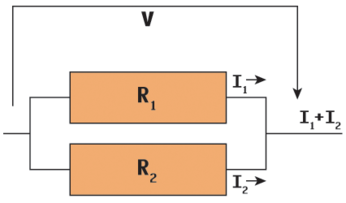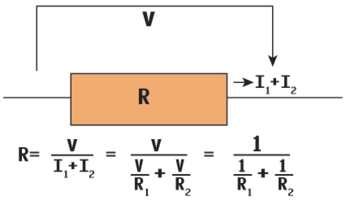# Programming Snapshot – SymPyArticle from Issue 248/2021

Whether he's filling the bathtub with water or routing electricity through resistors – Mike Schilli juggles mathematical formulas with the assistance of the Python SymPy package.

I still remember a traumatic event as an elementary school student: A weekend newspaper had set a logic puzzle for kids, the solution of which it promised to publish in the next issue a week later. It involved a bathtub with two taps: one of which filled the tub in 10 minutes, the other in 15. The question was how long would it take to fill the tub if both taps were turned on all the way.

As a little boy, I was absolutely sure that 10 plus 15 equals 25, which is 25 minutes. My father laughed and suggested that couldn't be true, because two taps would fill the bathtub faster than one alone. The next weekend, I was initially triumphant, because there it was – printed in black and white in the following issue – the confirmation that 25 minutes was the correct solution.

But disillusionment followed one week later: After receiving angry reader comments, the newspaper had to admit that it had made a mistake, because it does not take 25 minutes, but only 6 minutes, to fill the tub with both taps. I nearly fell off my chair and decided at that point to become a famous columnist peddling logic puzzles.

#### Faucets and Resistors

It was only much later, in college, during a lecture on circuit technology, that I came across a similar problem, which somehow translated nicely to a solution to the bathtub problem: parallel arrangement of resistors in a circuit. The electrical engineer measures the resistance of a conductor in ohms, and the higher the value, the more it slows down the flow of electrical current.

Now how do you calculate the equivalent resistance of a parallel circuit with two resistors R1 and R2 as shown in Figure 1? The solution involves the currents I1 and I2 flowing through each resistor. They add up to the total current I after the two branches are reunited. The measured voltage is the same everywhere, that is V, and according to Ohm's law V = R*I. Ergo, with individual currents I1 and I2 adding up to I, the result comes out as V/R1 + V/R2.Figure 1: Two resistors R1 and R2 in a parallel circuit …

If we now replace the two parallel resistors R1 and R2 with a substitute resistor R, we also get I = V/R for the total current, so this gets us: V/R = V/R1 + V/R2. The voltage V can be canceled out to give 1/R = 1/R1 + 1/R2 (Figure 2). After transformation, the following result appears:

R = (R1*R2)/(R1+R2)Figure 2: … and their equivalent resistance R.

Today, computer programs perform the computational work instead of the engineer doing it manually. Listing 1  shows the development of the formula using SymPy, a symbolic algebra package in Python. It can be used to define symbols, which the package later leaves intact when evaluating formulas, instead of immediately replacing variables with values and determining the formula's numerical result. With the `simplify()` function, SymPy can simplify expressions using the rules of algebra – just as any mathematically skilled person would do.

Listing 1

parallel.py

```01 #!/usr/bin/env python3
02 from sympy import simplify, symbols, pprint
03 r, r1, r2, v, i1, i2 = symbols("r r1 r2 v i1 i2")
04 i1 = v / r1
05 i2 = v / r2
06 r = v / (i1 + i2)
07 pprint(simplify(r))```

Thus, line 3 in Listing 1 defines a whole slew of symbols. For the two resistors of the circuit and their equivalent resistance, there are `r1`, `r2`, and `r`. For the applied voltage, there is `v`; for the two current components, there are `i1` and `i2`. The formulas in lines 4 to 6 apply Ohm's law and sum the partial currents to receive at the total current.

The resulting formula for the equivalent resistance `r` comes to light when the script is called using the `pprint()` (pretty print) function (Figure 3). SymPy has obviously realized that the voltage `v` can be canceled out of the fraction, and the result no longer depends on it.

If you use Python 3, you can install packages like sympy and matplotlib, which I'll be using in a moment to illustrate results, easily with the command:

`pip3 install <package>`

In order to avoid pulling the carpet out from under the feet of other Python scripts on the same host, I prefer doing that in Python's virtual environment .

#### From Circuit to Bathtub

This formula also works for the bathtub problem mentioned earlier: In the numerator, the filling times per tap get multiplied (10*15), and the denominator holds their sum (10+15). According to Adam Riese, this comes out to w150/25 (i.e., six minutes), just like in the example solution in the Sunday paper.

By the way, you can also think of the solution as follows: After one minute, the first tap has filled the tub to one tenth and the second to one fifteenth. Both together fill in one minute 1/10 + 1/15 = 3/30 + 2/30 = 5/30 = 1/6 of the tub, so you can get into the bath after six minutes.

#### Borderline

What happens if both resistors in the parallel circuit drop down to 0 ohms – that is, they do not slow down the current flow at all, or, in the case of a bathtub with Niagara Falls-style faucets, fill the tub in next to no time? Intuitively, it is clear that this kind of mega-faucet would fill the tub practically as fast when connected in parallel as when operated individually. But if you set the values for (R1*R2)/(R1+R2) in the formula (R1*R2)/(R2)/(R1+R2) to zero and pass the construct to a Python program for computing, you will experience a fatal error: Computers steadfastly refuse to perform divisions by zero, because it is mathematically undefined.

In the present case, however, both the numerator and the denominator contain a value tending towards zero, which sometimes yields interesting (because finite) results. Mind you, a true zero as denominator defies mathematical definition, but the limit for R1 and R2 approaching zero can definitely be computed.

SymPy provides the `limit()` function, which takes a symbolic formula, a symbol (such as `r1`) and a limit (here ` `). Listing 2 defines `r1 = r2` beforehand, meaning that both variables in the `limit()` function tend towards zero. The result for the equivalent resistor, as the values for `r1` and `r2` approach zero, is that the script returns, as expected, a value of zero (Listing 3).

Listing 2

limit.py

```01 #!/usr/bin/env python3
02 from sympy import limit, symbols
03 r1, r2, r = symbols("r1 r2 r")
04
05 r = (r1 * r2) / (r1 + r2)
06 r1 = r2
07
08 # r1/2->0
09 print(limit(r, r1, 0))
10
11 # 1/x with x->0
12 x = symbols("x")
13 print(limit(1/x, x, 0))```

Listing 3

Limit Value

```\$ ./limit.py
0
oo```

The second test case, starting from line 12 in Listing 2, illustrates with an example what happens to another formula, `1/x`, when `x` tends towards zero. The output in Listing 3 shows here that the result of the formula tends to infinity in this case, which is what the ASCII output `oo` tries to illustrate.

Express-Checkout as PDF
Price \$2.95
(incl. VAT)

SINGLE ISSUES

SUBSCRIPTIONS

## Related content

• MathLex

MathLex lets you easily transform handwritten math formulas to digital format and use them on the web.

• Hard Disk Dashboard

To keep an eye on the remaining disk space during storage-intensive operations, you can check out this speedometer/odometer written in Go.

• Chip Shot

We all know that the Fyne framework for Go can be used to create GUIs for the desktop, but you can also write games with it. Mike Schilli takes on a classic from the soccer field.

• Maxima

This free algebra tool helps you keep ahead of the calculations.

• Gnumeric

OpenOffice Calc is fine for most spreadsheet applications, but if you’re looking for better performance and a smaller footprint, try Calc’s lean competitor, Gnumeric.

# Support Our Work

Linux Magazine content is made possible with support from readers like you. Please consider contributing when you've found an article to be beneficial.

## News

• ### The GNU Project Celebrates Its 40th Birthday

September 27 marks the 40th anniversary of the GNU Project, and it was celebrated with a hacker meeting in Biel/Bienne, Switzerland.

• ### Linux Kernel Reducing Long-Term Support

LTS support for the Linux kernel is about to undergo some serious changes that will have a considerable impact on the future.

• ### Fedora 39 Beta Now Available for Testing

For fans and users of Fedora Linux, the first beta of release 39 is now available, which is a minor upgrade but does include GNOME 45.

• ### Fedora Linux 40 to Drop X11 for KDE Plasma

When Fedora 40 arrives in 2024, there will be a few big changes coming, especially for the KDE Plasma option.

• ### Real-Time Ubuntu Available in AWS Marketplace

Anyone looking for a Linux distribution for real-time processing could do a whole lot worse than Real-Time Ubuntu.

• ### KSMBD Finally Reaches a Stable State

For those who've been looking forward to the first release of KSMBD, after two years it's no longer considered experimental.

• ### Nitrux 3.0.0 Has Been Released

The latest version of Nitrux brings plenty of innovation and fresh apps to the table.

• ### Linux From Scratch 12.0 Now Available

If you're looking to roll your own Linux distribution, the latest version of Linux From Scratch is now available with plenty of updates.

• ### Linux Kernel 6.5 Has Been Released

The newest Linux kernel, version 6.5, now includes initial support for two very exciting features.

• ### UbuntuDDE 23.04 Now Available

A new version of the UbuntuDDE remix has finally arrived with all the updates from the Deepin desktop and everything that comes with the Ubuntu 23.04 base.